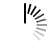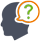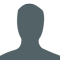Diwali Bonanza | Get 30-Days Free Fun-Filled Learning | Subscribe Now1800-833-6464 Buy Activation Why Tutorix Features Login

#DoubtTushar

In figure below, $DE\ ∥\ BC$ such that $AE\ =\ (\frac{1}{4})AC$. If $AB\ =\ 6\ cm$, find $AD$.Solutions :Akhilesh

Given:

In the given figure, $DE\ ∠¥\ BC$ such that $AE\ =\ (\frac{1}{4})AC$ and $AB\ =\ 6\ cm$.

To do:

We have to find $AD$.

Solution:

In $\vartriangle ADE$ and $\vartriangle ABC$,

$\angle A = \angle A$  (Common)

$\angle ADE = \angle ABC$ ($AB||QR$, Corresponding angles)

Therefore,

$\vartriangle ADE ∠¼ \vartriangle ABC$  (By AA similarity)

$\frac{AD}{AB} = \frac{AE}{AC}$  (Corresponding parts of similar triangles are proportional)

$\frac{AD}{6} = \frac{1}{4}$   ($AE\ =\ (\frac{1}{4})AC$, this implies, $\frac{AE}{AC} =\frac{1}{4}$)

$AD = \frac{6}{4}$

$AD = 1.5\ cm$

The measure of $AD$ is $1.5\ cm$.

0

﻿